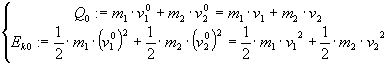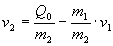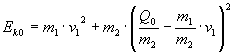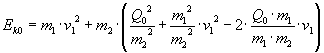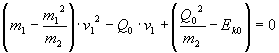Bouncing balls, geometry and physics

The core of the question is calculating the new speed vectors, after having stated two balls are bouncing together.

The key is working along the line joining the centers of the balls. I’ll consider here the case of the same masses (set to unitary value).

The stuff is arranged with following steps.

1) Are they touching?

 Trivial: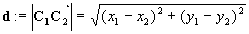They’re touching iff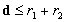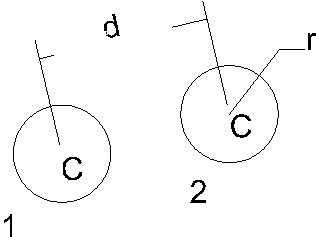2) Find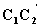direction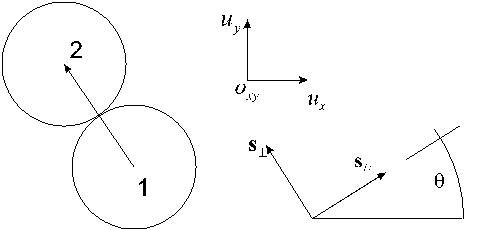Ok, they’re touching. We’ll need the direction ofvector, the line joining the balls centers (with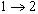as positive direction). So, let’s find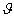(i.e.: cosine and sine).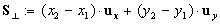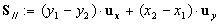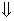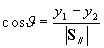3) Find the speed components along the centers line

Now we have the angle, we can find the speed components on the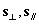system.

For a single ball: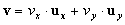Using the rotation equations: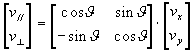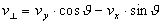4) Apply physics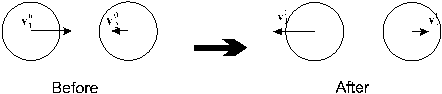Now it’s easy to apply the physics of the system to the calculated components:

The // components don’t vary. For the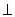components: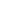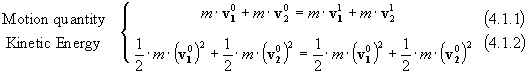Setting: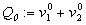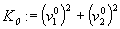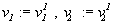We have: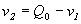from (4.1.1)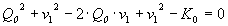from (4.1.2)

And also: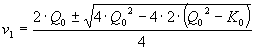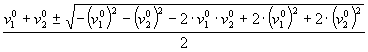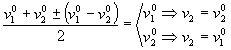The first solution refers to the case of balls not crashing, the second, the meaning one, says the speeds are exchanged.

4.1) Signs checking

After having stated the balls are touching we need also a check about the signs of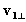and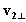: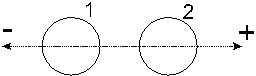++ They’re really crashing together (if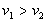)

+- Crashing

-- Crashing (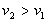)

-+ Moving away

(In fact, in an animation program we also have to track the crash event in order to avoid its continuos firing when balls are near enough)

5) Back to ordinary Cartesian space

When you have newspeed components, and therefore new speed vectors, expressed in terms ofand // components, you have to back to the Oxy coordinates system, with the rotation: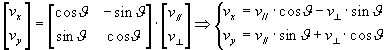Resume:

1. Touch checking
2. Find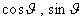3. Find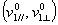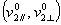4. Finds new components
1. Checks for signs
2. Find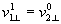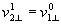and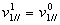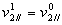That is:swaps and // remain the same
5. Back to Oxy and get new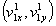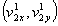Appendix: A brief view about the case of different masses